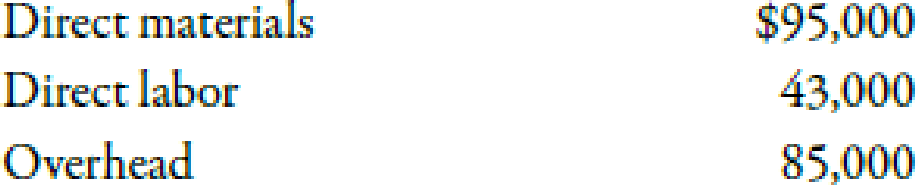Chapter 8, Problem 64P### Managerial Accounting: The Corners...

7th Edition
Maryanne M. Mowen + 2 others
ISBN: 9781337115773

#### Solutions

Chapter
Section### Managerial Accounting: The Corners...

7th Edition
Maryanne M. Mowen + 2 others
ISBN: 9781337115773
Textbook Problem
5 views

# Sell or Process Further, Basic AnalysisShenista Inc. produces four products (Alpha, Beta, Gamma, and Delta) from a common input. The joint costs for a typical quarter follow:The revenues from each product are as follows: Alpha, $100,000; Beta,$93,000; Gamma, $30,000; and Delta,$40,000.Management is considering processing Delta beyond the split-off point, which would increase the sales value of Delta to $75,000. However, to process Delta further means that the company must rent some special equipment that costs$15,400 per quarter. Additional materials and labor also needed will cost $8,500 per quarter.Required: 1. What is the operating profit earned by the four products for one quarter? 2. CONCEPTUAL CONNECTION Should the division process Delta further or sell it at split-off? What is the effect of the decision on quarterly operating profit? 1. To determine Calculate the amount of operating profit earned by products for one quarter. Explanation Sell-or-Process-Further Decision: Sell-or-process further decision can be defined as the analysis of relevant costing which emphasizes on decision making regarding whether to process the product beyond its split-off point. Use the following formula to calculate the amount of operating profit: Operating profit=Sales1Costs2 Substitute$263,000 for sales and $223,000 for costs in the above formula. Operating profit=$263,000$223,000=$40,000

Therefore, the amount of operating profit is \$40,000.

Working Notes:

1

2.

To determine

Describe whether Delta should be processed further or sold at split-off. Also, describe the effect on quarterly operating profit.

### Still sussing out bartleby?

Check out a sample textbook solution.

See a sample solution

#### The Solution to Your Study Problems

Bartleby provides explanations to thousands of textbook problems written by our experts, many with advanced degrees!

Get Started

#### Find more solutions based on key concepts